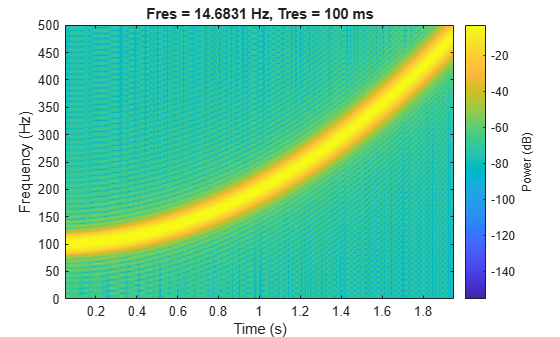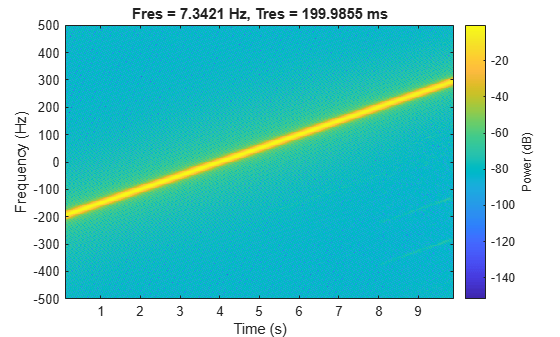# chirp

Swept-frequency cosine

## Syntax

``y = chirp(t,f0,t1,f1)``
``y = chirp(t,f0,t1,f1,method)``
``y = chirp(t,f0,t1,f1,method,phi)``
``y = chirp(t,f0,t1,f1,'quadratic',phi,shape)``
``y = chirp(___,cplx)``

## Description

example

````y = chirp(t,f0,t1,f1)` generates samples of a linear swept-frequency cosine signal at the time instances defined in array `t`. The instantaneous frequency at time 0 is `f0` and the instantaneous frequency at time `t1` is `f1`.```

example

````y = chirp(t,f0,t1,f1,method)` specifies an alternative sweep `method` option.```

example

````y = chirp(t,f0,t1,f1,method,phi)` specifies the initial phase.```

example

````y = chirp(t,f0,t1,f1,'quadratic',phi,shape)` specifies the shape of the spectrogram of a quadratic swept-frequency signal.```

example

````y = chirp(___,cplx)` returns a real chirp if `cplx` is specified as `'real'` and returns a complex chirp if `cplx` is specified as `'complex'`.```

## Examples

collapse all

Generate a chirp with linear instantaneous frequency deviation. The chirp is sampled at 1 kHz for 2 seconds. The instantaneous frequency is 0 at t = 0 and crosses 250 Hz at t = 1 second.

```t = 0:1/1e3:2; y = chirp(t,0,1,250);```

Compute and plot the spectrogram of the chirp. Divide the signal into segments such that the time resolution is 0.1 second. Specify 99% of overlap between adjoining segments and a spectral leakage of 0.85.

```pspectrum(y,1e3,'spectrogram','TimeResolution',0.1, ... 'OverlapPercent',99,'Leakage',0.85)```Generate a chirp with quadratic instantaneous frequency deviation. The chirp is sampled at 1 kHz for 2 seconds. The instantaneous frequency is 100 Hz at t = 0 and crosses 200 Hz at t = 1 second.

```t = 0:1/1e3:2; y = chirp(t,100,1,200,'quadratic');```

Compute and plot the spectrogram of the chirp. Divide the signal into segments such that the time resolution is 0.1 second. Specify 99% of overlap between adjoining segments and a spectral leakage of 0.85.

```pspectrum(y,1e3,'spectrogram','TimeResolution',0.1, ... 'OverlapPercent',99,'Leakage',0.85)```Generate a convex quadratic chirp sampled at 1 kHz for 2 seconds. The instantaneous frequency is 400 Hz at t = 0 and crosses 300 Hz at t = 1 second.

```t = 0:1/1e3:2; fo = 400; f1 = 300; y = chirp(t,fo,1,f1,'quadratic',[],'convex');```

Compute and plot the spectrogram of the chirp. Divide the signal into segments such that the time resolution is 0.1 second. Specify 99% of overlap between adjoining segments and a spectral leakage of 0.85.

```pspectrum(y,1e3,'spectrogram','TimeResolution',0.1, ... 'OverlapPercent',99,'Leakage',0.85)```Generate a concave quadratic chirp sampled at 1 kHz for 4 seconds. Specify the time vector so that the instantaneous frequency is symmetric about the halfway point of the sampling interval, with a minimum frequency of 100 Hz and a maximum frequency of 500 Hz.

```t = -2:1/1e3:2; fo = 100; f1 = 200; y = chirp(t,fo,1,f1,'quadratic',[],'concave');```

Compute and plot the spectrogram of the chirp. Divide the signal into segments such that the time resolution is 0.1 second. Specify 99% of overlap between adjoining segments and a spectral leakage of 0.85.

```pspectrum(y,t,'spectrogram','TimeResolution',0.1, ... 'OverlapPercent',99,'Leakage',0.85)```Generate a logarithmic chirp sampled at 1 kHz for 10 seconds. The instantaneous frequency is 10 Hz initially and 400 Hz at the end.

```t = 0:1/1e3:10; fo = 10; f1 = 400; y = chirp(t,fo,10,f1,'logarithmic');```

Compute and plot the spectrogram of the chirp. Divide the signal into segments such that the time resolution is 0.2 second. Specify 99% of overlap between adjoining segments and a spectral leakage of 0.85.

```pspectrum(y,t,'spectrogram','TimeResolution',0.2, ... 'OverlapPercent',99,'Leakage',0.85)```Use a logarithmic scale for the frequency axis. The spectrogram becomes a line, with high uncertainty at low frequencies.

```ax = gca; ax.YScale = 'log';```Generate a complex linear chirp sampled at 1 kHz for 10 seconds. The instantaneous frequency is –200 Hz initially and 300 Hz at the end. The initial phase is zero.

```t = 0:1/1e3:10; fo = -200; f1 = 300; y = chirp(t,fo,t(end),f1,'linear',0,'complex');```

Compute and plot the spectrogram of the chirp. Divide the signal into segments such that the time resolution is 0.2 second. Specify 99% of overlap between adjoining segments and a spectral leakage of 0.85.

```pspectrum(y,t,'spectrogram','TimeResolution',0.2, ... 'OverlapPercent',99,'Leakage',0.85)```Verify that a complex chirp has real and imaginary parts that are equal but with ${90}^{\circ }$ phase difference.

```x = chirp(t,fo,t(end),f1,'linear',0) + 1j*chirp(t,fo,t(end),f1,'linear',-90); pspectrum(x,t,'spectrogram','TimeResolution',0.2, ... 'OverlapPercent',99,'Leakage',0.85)```## Input Arguments

collapse all

Time array, specified as a vector.

Data Types: `single` | `double`

Initial instantaneous frequency at time 0, specified as a real scalar expressed in Hz.

Data Types: `single` | `double`

Reference time, specified as a positive scalar expressed in seconds.

Data Types: `single` | `double`

Instantaneous frequency at time `t1`, specified as a real scalar expressed in Hz.

Data Types: `single` | `double`

Sweep method, specified as `'linear'`, `'quadratic'`, or `'logarithmic'`.

• `'linear'` — Specifies an instantaneous frequency sweep fi(t) given by

`${f}_{i}\left(t\right)={f}_{0}+\beta t,$`

where

`$\beta =\left({f}_{1}-{f}_{0}\right)/{t}_{1}$`

and the default value for f0 is 0. The coefficient β ensures that the desired frequency breakpoint f1 at time t1 is maintained.

• `'quadratic'` — Specifies an instantaneous frequency sweep fi(t) given by

`${f}_{i}\left(t\right)={f}_{0}+\beta {t}^{2},$`

where

`$\beta =\left({f}_{1}-{f}_{0}\right)/{t}_{1}{}^{2}$`

and the default value for f0 is 0. If f0 > f1 (downsweep), the default shape is convex. If ff1 (upsweep), the default shape is concave.

• `'logarithmic'` — Specifies an instantaneous frequency sweep fi(t) given by

`${f}_{i}\left(t\right)={f}_{0}×{\beta }^{t},$`

where

`$\beta ={\left(\frac{{f}_{1}}{{f}_{0}}\right)}^{\frac{1}{{t}_{1}}}$`

and the default value for f0 is 10–6.

Initial phase, specified as a positive scalar expressed in degrees.

Data Types: `single` | `double`

Spectrogram shape of quadratic chirp, specified as `'convex'` or `'concave'`. `shape` describes the shape of the parabola with respect to the positive frequency axis. If not specified, `shape` is `'convex'` for the downsweep case with f0 > f1, and `'concave'` for the upsweep case with f0 < f1.Output complexity, specified as `'real'` or `'complex'`.

## Output Arguments

collapse all

Swept-frequency cosine signal, returned as a vector.

## Version History

Introduced before R2006a